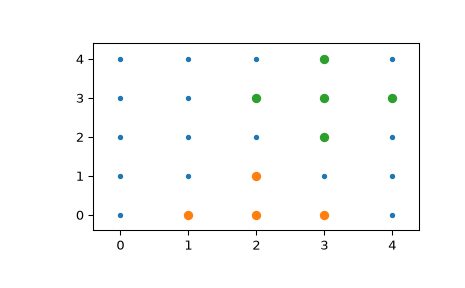# scipy.spatial.KDTree.query_ball_point¶

KDTree.query_ball_point(self, x, r, p=2.0, eps=0)[source]

Find all points within distance r of point(s) x.

Parameters
xarray_like, shape tuple + (self.m,)

The point or points to search for neighbors of.

rpositive float

The radius of points to return.

pfloat, optional

Which Minkowski p-norm to use. Should be in the range [1, inf].

epsnonnegative float, optional

Approximate search. Branches of the tree are not explored if their nearest points are further than r / (1 + eps), and branches are added in bulk if their furthest points are nearer than r * (1 + eps).

Returns
resultslist or array of lists

If x is a single point, returns a list of the indices of the neighbors of x. If x is an array of points, returns an object array of shape tuple containing lists of neighbors.

Notes

If you have many points whose neighbors you want to find, you may save substantial amounts of time by putting them in a KDTree and using query_ball_tree.

Examples

>>> from scipy import spatial
>>> x, y = np.mgrid[0:5, 0:5]
>>> points = np.c_[x.ravel(), y.ravel()]
>>> tree = spatial.KDTree(points)
>>> sorted(tree.query_ball_point([2, 0], 1))
[5, 10, 11, 15]


Query multiple points and plot the results:

>>> import matplotlib.pyplot as plt
>>> points = np.asarray(points)
>>> plt.plot(points[:,0], points[:,1], '.')
>>> for results in tree.query_ball_point(([2, 0], [3, 3]), 1):
...     nearby_points = points[results]
...     plt.plot(nearby_points[:,0], nearby_points[:,1], 'o')
>>> plt.margins(0.1, 0.1)
>>> plt.show()#### Previous topic

scipy.spatial.KDTree.query

#### Next topic

scipy.spatial.KDTree.query_ball_tree